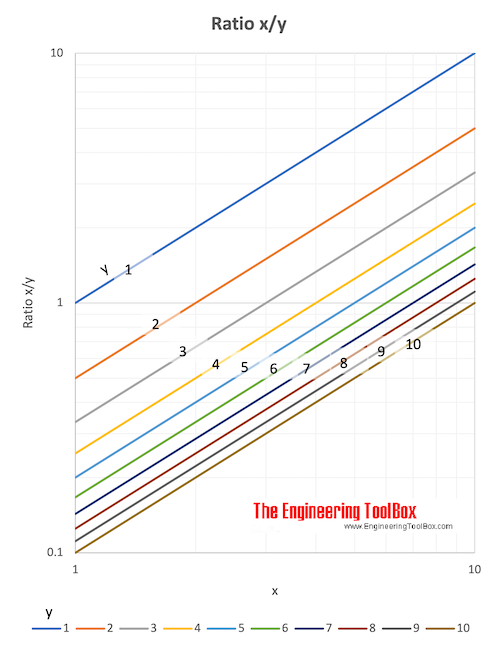Engineering ToolBox - Resources, Tools and Basic Information for Engineering and Design of Technical Applications!

# Ratios and Proportions

## The relative values between quantities - ratios and proportions.

### Ratio

The ratio of one quantity to an other is a fraction - and the number of times one quantity is contained in another quantity of the same kind.

The ratio between two quantities is the quotient obtained by dividing the first quantity by the second.

#### Example - Ratio

The ratio between 3 and 6 can be expressed as

3 / 6 = 1 / 2 = 0.5

Ratio can also be indicated by the sign ":" - like 3 : 6 or 1 : 2.### Reciprocal or Inverse Ratio

The reciprocal or inverse ratio is the opposite of the original ratio.

#### Example the Inverse Ratio

The inverse ratio between 6 and 3 can be expressed as

6 : 3  = 2 : 1 = 2

### Proportion

Proportion is the equality of ratios.

1 : 2 = 3 : 6

## Related Topics

• Basics - The SI-system, unit converters, physical constants, drawing scales and more.
• Mathematics - Mathematical rules and laws - numbers, areas, volumes, exponents, trigonometric functions and more.

## Engineering ToolBox - SketchUp Extension - Online 3D modeling!

Add standard and customized parametric components - like flange beams, lumbers, piping, stairs and more - to your Sketchup model with the Engineering ToolBox - SketchUp Extension - enabled for use with the amazing, fun and free SketchUp Make and SketchUp Pro .Add the Engineering ToolBox extension to your SketchUp from the SketchUp Pro Sketchup Extension Warehouse!

Translate

## Privacy

We don't collect information from our users. Only emails and answers are saved in our archive. Cookies are only used in the browser to improve user experience.

Some of our calculators and applications let you save application data to your local computer. These applications will - due to browser restrictions - send data between your browser and our server. We don't save this data.

## Citation

• Engineering ToolBox, (2007). Ratios and Proportions. [online] Available at: https://www.engineeringtoolbox.com/ratio-proportion-d_1300.html [Accessed Day Mo. Year].

Modify access date.

. .

#### Scientific Online Calculator6 24